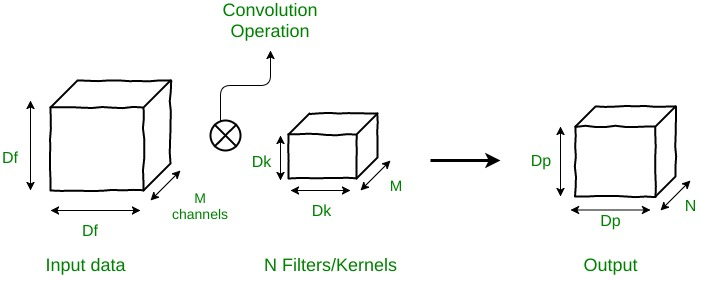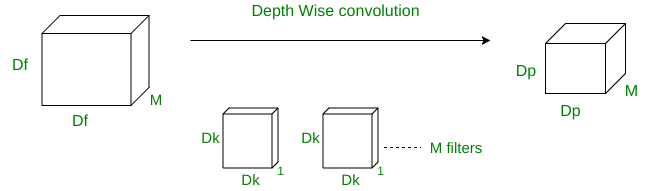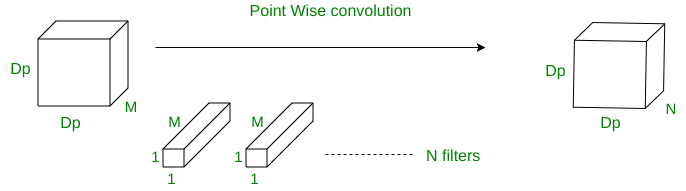Related Articles

# Depth wise Separable Convolutional Neural Networks

• Difficulty Level : Medium
• Last Updated : 28 Aug, 2019

Convolution is a very important mathematical operation in artificial neural networks(ANN’s). Convolutional neural networks (CNN’s) can be used to learn features as well as classify data with the help of image frames. There are many types of CNN’s. One class of CNN’s are depth wise separable convolutional neural networks.

These type of CNN’s are widely used because of the following two reasons –

• They have lesser number of parameters to adjust as compared to the standard CNN’s, which reduces overfitting
• They are computationally cheaper because of fewer computations which makes them suitable for mobile vision applications

Some important applications of these type of CNN’s are MobileNet, Xception ( both proposed by Google )

This article explains the architecture and operations used by depth wise separable convolutional networks and derives its efficiency over simple convolution neural networks.

### Understanding Normal Convolution operation

Suppose there is an input data of size Df x Df x M, where Df x Df can be the image size and M is the number of channels (3 for an RGB image). Suppose there are N filters/kernels of size Dk x Dk x M. If a normal convolution operation is done, then, the output size will be Dp x Dp x N.The number of multiplications in 1 convolution operation = size of filter = Dk x Dk x M

Since there are N filters and each filter slides vertically and horizontally Dp times,

the total number of multiplications become N x Dp x Dp x (Multiplications per convolution)

So for normal convolution operation

Total no of multiplications = N x Dp2 x Dk2 x M

### Depth-Wise Separable Convolutions

Now look at depth-wise separable convolutions. This process is broken down into 2 operations –

1. Depth-wise convolutions
2. Point-wise convolutions

1. DEPTH WISE CONVOLUTION

In depth-wise operation, convolution is applied to a single channel at a time unlike standard CNN’s in which it is done for all the M channels. So here the filters/kernels will be of size Dk x Dk x 1. Given there are M channels in the input data, then M such filters are required. Output will be of size Dp x Dp x M.Cost of this operation:

A single convolution operation require Dk x Dk multiplications.

Since the filter are slided by Dp x Dp times across all the M channels,

the total number of multiplications is equal to M x Dp x Dp x Dk x Dk

So for depth wise convolution operation

Total no of multiplications = M x Dk2 x Dp2

2. POINT WISE CONVOLUTION

In point-wise operation, a 1×1 convolution operation is applied on the M channels. So the filter size for this operation will be 1 x 1 x M. Say we use N such filters, the output size becomes Dp x Dp x N.Cost of this operation:

A single convolution operation require 1 x M multiplications.

Since the filter is being slided by Dp x Dp times,

the total number of multiplications is equal to M x Dp x Dp x (no. of filters)

So for point wise convolution operation

Total no of multiplications = M x Dp2 x N

Therefore, for overall operation:

Total multiplications = Depth wise conv. multiplications + Point wise conv. multiplications

Total multiplications = M * Dk2 * Dp2 + M * Dp2 * N = M * Dp2 * (Dk2 + n)

So for depth wise separable convolution operation

Total no of multiplications = M x Dp2 x (Dk2 + N)

### Comparison between the complexities of these types of convolution operations

<

#### M x Dp2 x (Dk2 + N)

```  Complexity of depth wise separable convolutions
--------------------------------------------------   =  RATIO ( R )
Complexity of standard convolution
```

Upon solving:

Ratio(R) = 1/N + 1/Dk2

As an example, consider N = 100 and Dk = 512. Then the ratio R = 0.010004

This means that the depth wise separable convolution network, in this example, performs 100 times lesser multiplications as compared to a standard constitutional neural network.

This implies that we can deploy faster convolution neural network models without losing much of the accuracy.My Personal Notes arrow_drop_up